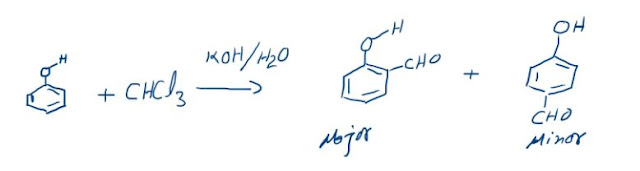## Electrochemical Series

Electrochemical Series , Many half cell potential values can be calculated keeping hydrogen electrode as the standard. These half cell potential values are standard values and are represented as the standard reduction potential values as shown in the following table which is also called “Electrochemical Series”. By measuring the potentials of various electrodes versus stand­ard hydrogen electrode (SHE), a series of standard electrode potentials has been established called Electrochemical Series.

## STANDARD REDUCTION POTENTIALS

 Element Electrode Reaction (Reduction) Standard Electrode Reduction potential Eo, volt Li Li+ + e- →  Li -3.05 K K+ + e- → K -2.925 Ca Ca2+ + 2e- → Ca -2.87 Na Na+ + e- → Na -2.714 Mg Mg2+ + 2e- → Mg -2.37 Al Al3+ + 3e- → Al -1.66 Zn Zn2+ + 2e- → Zn -0.7628 Cr Cr3+ + 3e- → Cr -0.74 Fe Fe2+ + 2e- → Fe -0.44 Cd Cd2+ + 2e- → Cd -0.403 Ni Ni2+ + 2e- → Ni -0.25 Sn Sn2+ + 2e- → Sn -0.14 H2 2H+ + 2e- → H2 0.00 Cu Cu2+ + 2e- → Cu +0.337 I2 I2 + 2e- → 2I- +0.535 Ag Ag+ + e- → Ag +0.799 Hg Hg2+ + 2e- → Hg +0.885 Br2 Br2 + 2e- → 2Br- +1.08 Cl2 Cl2 + 2e- → 2Cl- +1.36 Au Au3+ + 3e- → Au +1.50 F2 F2 + 2e- → 2F- +2.87

The negative sign of standard reduction potential indicates that an electrode when joined with SHE acts as anode and oxidation occurs on this electrode. Similarly, the positive sign of standard reduction potential indicates that the electrode when joined with SHE acts as cathode and reduction occurs on this electrode.

## Application of Electrochemical series

Some of the important applications of electrochemical series are discussed as follows:

### 1. Relative Oxidising and Reducing Powers of Various Substances/Reactivity of Metals.

The activity of the metal depends on its tendency to lose electron or electrons, i.e., tendency to form cation (M"+). This tendency depends on the magnitude of standard reduction potential. The metal which has high negative value (or smaller positive value) of standard reduction potential readily loses the electron or electrons and is converted into cation. Such a metal is said to be chemically active.
The chemical reactivity of metals decreases from top to bottom in the series. The metal higher in the series is more active than the metal lower in the series. For example F2 has highest reduction potential which means it is most easily reduced to F– ions. In other words, F2 is best oxidising agent. Li+ ion, on the other hand, had lowest reduction potential. Hence Li+ is weakest reducing agent or conversely Li metal is best reducing agent. Thus, it can be concluded that substances with higher reduction potentials are strong oxidising agents while substances with lower reduction potentials are strong reducing agents.

### 2. Calculation of Standard EMF of the cell

Standard EMF of the cell can be calculated by applying the formula

E cell = Eº (cathode) + Eº (anode)

The electrode with higher electrode potential (Eº) act as cathode while that with lower electrode potential will act as anode.

### 3. Predicting Feasibility of Redox Reaction.

Electrochemical series helps to predict the feasibility of the redox reaction in a given direction. If Ecell is positive then the redox reaction is feasible otherwise it is non-feasible.

### 4. Predicting whether a Metal can Displace H2 Gas from Acid or not.  The chemical reaction between metal M and acid to liberate H2 gas is represented by the reaction.

M (s)           Mn+   + ne

2H+    + 2e          H2

All metals lying above hydrogen in electrochemical series can liberate H2 gas by reaction with acids. On the other hand, the metal lying below hydrogen in the electrochemical series cannot undergo such a reaction.

### The Reimer-Tiemann reaction: Reaction and its Mechanism

Reimer-Tiemann Reaction Phenols on reaction with chloroform in the presence of sodium hydroxide (or potassium hydroxide) solution give hydro...